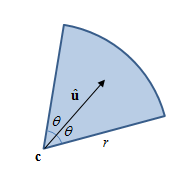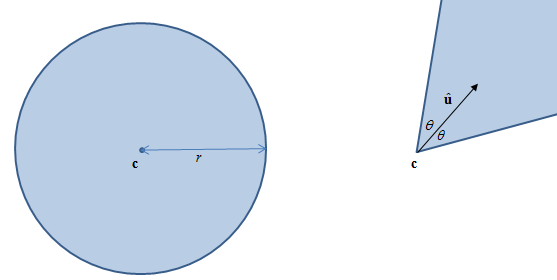# 面试题：检测点是否在扇形之内## 问题分析### 距离

\mathbf{p}\mathbf{c} 的距离小于 r， 用数学方式表示:

|\mathbf{p} - \mathbf{c}| < r

### 极坐标

\begin{align*} \phi &= \mathrm{atan2}(p_y - c_y, p_x - c_x)\\ \alpha &= \mathrm{atan2}(u_y, u_x) \end{align*}

\begin{align*} \alpha_1 &< \phi - 2\pi < \alpha_2 \text{ ; or}\\ \alpha_1 &< \phi < \alpha_2 \text{ ; or}\\ \alpha_1 &< \phi + 2\pi < \alpha_2 \end{align*}

### 点积

\cos^{-1}\left (\mathbf{\frac{p-c}{|p-c|}} \cdot \mathbf{\hat{u}} \right\) < \theta

## 编码与优化

// Naive
bool IsPointInCircularSector(
float cx, float cy, float ux, float uy, float r, float theta,
float px, float py)
{
assert(cosTheta > -1 && cosTheta < 1);
assert(squaredR > 0.0f);

// D = P - C
float dx = px - cx;
float dy = py - cy;

// |D| = (dx^2 + dy^2)^0.5
float length = sqrt(dx * dx + dy * dy);

// |D| > r
if (length > r)
return false;

// Normalize D
dx /= length;
dy /= length;

// acos(D dot U) < theta
return acos(dx * ux + dy * uy) < theta;
}


### 优化版本1: 基本优化

|\mathbf{p - c}|^2 < r^2

\frac{\mathbf{p-c}}{|\mathbf{p-c}|} \cdot \mathbf{\hat{u}} > \cos \theta

(\mathbf{p-c}) \cdot \mathbf{\hat{u}} > \mathbf{|p-c|} \cos \theta


// Basic: use squareR and cosTheta as parameters, defer sqrt(), eliminate division
bool IsPointInCircularSector1(
float cx, float cy, float ux, float uy, float squaredR, float cosTheta,
float px, float py)
{
assert(cosTheta > -1 && cosTheta < 1);
assert(squaredR > 0.0f);

// D = P - C
float dx = px - cx;
float dy = py - cy;

// |D|^2 = (dx^2 + dy^2)
float squaredLength = dx * dx + dy * dy;

// |D|^2 > r^2
if (squaredLength > squaredR)
return false;

// |D|
float length = sqrt(squaredLength);

// D dot U > |D| cos(theta)
return dx * ux + dy * uy > length * cosTheta;
}


### 优化版本2: 去除开平方

(\mathbf{(p-c)} \cdot u)^2 > {|p-c|}^2 \cos^2 \theta \qquad \textrm{Wrong!}

1. 若左侧为非负数，右侧为非负数，检测 ((\mathbf{p-c}) \cdot \mathbf{\hat{u}})^2 > {|\mathbf{p-c}|}^2 \cos^2 \theta
2. 若左侧为为负数，右侧为负数，检测 ((\mathbf{p-c}) \cdot \mathbf{\hat{u}})^2 < {|\mathbf{p-c}|}^2 \cos^2 \theta
3. 若左侧为非负数，右侧为负数，那么不等式(2.3)一定成立
4. 若左侧为负数，右侧为非负数，那么不等式(2.3)一定不成立


// Eliminate sqrt()
bool IsPointInCircularSector2(
float cx, float cy, float ux, float uy, float squaredR, float cosTheta,
float px, float py)
{
assert(cosTheta > -1 && cosTheta < 1);
assert(squaredR > 0.0f);

// D = P - C
float dx = px - cx;
float dy = py - cy;

// |D|^2 = (dx^2 + dy^2)
float squaredLength = dx * dx + dy * dy;

// |D|^2 > r^2
if (squaredLength > squaredR)
return false;

// D dot U
float DdotU = dx * ux + dy * uy;

// D dot U > |D| cos(theta)
// <=>
// (D dot U)^2 > |D|^2 (cos(theta))^2 if D dot U >= 0 and cos(theta) >= 0
// (D dot U)^2 < |D|^2 (cos(theta))^2 if D dot U <  0 and cos(theta) <  0
// true                               if D dot U >= 0 and cos(theta) <  0
// false                              if D dot U <  0 and cos(theta) >= 0
if (DdotU >= 0 && cosTheta >= 0)
return DdotU * DdotU > squaredLength * cosTheta * cosTheta;
else if (DdotU < 0 && cosTheta < 0)
return DdotU * DdotU < squaredLength * cosTheta * cosTheta;
else
return DdotU >= 0;
}


### 优化版本3: 减少比较


// Bit trick
bool IsPointInCircularSector3(
float cx, float cy, float ux, float uy, float squaredR, float cosTheta,
float px, float py)
{
assert(cosTheta > -1 && cosTheta < 1);
assert(squaredR > 0.0f);

// D = P - C
float dx = px - cx;
float dy = py - cy;

// |D|^2 = (dx^2 + dy^2)
float squaredLength = dx * dx + dy * dy;

// |D|^2 > r^2
if (squaredLength > squaredR)
return false;

// D dot U
float DdotU = dx * ux + dy * uy;

// D dot U > |D| cos(theta)
// <=>
// (D dot U)^2 > |D|^2 (cos(theta))^2 if D dot U >= 0 and cos(theta) >= 0
// (D dot U)^2 < |D|^2 (cos(theta))^2 if D dot U <  0 and cos(theta) <  0
// true                               if D dot U >= 0 and cos(theta) <  0
// false                              if D dot U <  0 and cos(theta) >= 0
union {
float f;
unsigned u;
}a, b, lhs, rhs;
a.f = DdotU;
b.f = cosTheta;
unsigned asign = a.u & cSignMask;
unsigned bsign = b.u & cSignMask;
if (asign == bsign) {
lhs.f = DdotU * DdotU;
rhs.f = squaredLength * cosTheta * cosTheta;
lhs.u |= asign;
rhs.u |= asign;
return lhs.f > rhs.f;
}
else
return asign == 0;
}



const unsigned N = 1000;
const unsigned M = 100000;

template <bool naiveParam, typename TestFunc>
float Test(TestFunc f, float rmax = 2.0f) {
unsigned count = 0;
for (int i = 0; i < N; i++) {
float cx = gCx[i];
float cy = gCy[i];
float ux = gUx[i];
float uy = gUy[i];
float r = naiveParam ? gR[i] : gSquaredR[i];
float t = naiveParam ? gTheta[i] : gCosTheta[i];

for (int j = 0; j < M; j++) {
if (f(cx, cy, ux, uy, r, t, gPx[j], gPy[j]))
count++;
}
}
return (float)count / (N * M);
}



NoOperation hit=0% time=0.376s
IsPointInCircularSector hit=30.531% time=3.964s

NoOperation hit=0% time=0.364s
IsPointInCircularSector1 hit=30.531% time=0.643s
IsPointInCircularSector2 hit=30.531% time=0.614s
IsPointInCircularSector3 hit=30.531% time=0.571s


NoOperation是指测试的函数只简单传回false，用来检测函循环、读取测试数据和函数调用的开销。之后测的时间会减去这个开销。


NoOperation hit=0% time=0.453s
IsPointInCircularSector hit=30.531% time=2.645s

NoOperation hit=0% time=0.401s
IsPointInCircularSector1 hit=30.531% time=0.29s
IsPointInCircularSector2 hit=30.531% time=0.32s
IsPointInCircularSector3 hit=30.531% time=0.455s


### 优化版本4和5: SOA SIMD

// SSE2, SOA(struct of array) layout
// SSE2, SOA(struct of array) layout
__m128 IsPointInCircularSector4(
__m128 cx, __m128 cy, __m128 ux, const __m128& uy, const __m128& squaredR, const __m128& cosTheta,
const __m128& px, const __m128& py)
{
// D = P - C
__m128 dx = _mm_sub_ps(px, cx);
__m128 dy = _mm_sub_ps(py, cy);

// |D|^2 = (dx^2 + dy^2)
__m128 squaredLength = _mm_add_ps(_mm_mul_ps(dx, dx), _mm_mul_ps(dy, dy));

// |D|^2 < r^2
__m128 lengthResult = _mm_cmpgt_ps(squaredR, squaredLength);

// |D|
__m128 length = _mm_sqrt_ps(squaredLength);

// D dot U
__m128 DdotU = _mm_add_ps(_mm_mul_ps(dx, ux), _mm_mul_ps(dy, uy));

// D dot U > |D| cos(theta)
__m128 angularResult = _mm_cmpgt_ps(DdotU, _mm_mul_ps(length, cosTheta));

__m128 result = _mm_and_ps(lengthResult, angularResult);

return result;
}

// SSE2, SOA(struct of array) layout without sqrt()
__m128 IsPointInCircularSector5(
__m128 cx, __m128 cy, __m128 ux, const __m128& uy, const __m128& squaredR, const __m128& cosTheta,
const __m128& px, const __m128& py)
{
// D = P - C
__m128 dx = _mm_sub_ps(px, cx);
__m128 dy = _mm_sub_ps(py, cy);

// |D|^2 = (dx^2 + dy^2)
__m128 squaredLength = _mm_add_ps(_mm_mul_ps(dx, dx), _mm_mul_ps(dy, dy));

// |D|^2 < r^2
__m128 lengthResult = _mm_cmpgt_ps(squaredR, squaredLength);

// D dot U
__m128 DdotU = _mm_add_ps(_mm_mul_ps(dx, ux), _mm_mul_ps(dy, uy));

// D dot U > |D| cos(theta)
// <=>
// (D dot U)^2 > |D|^2 (cos(theta))^2 if D dot U >= 0 and cos(theta) >= 0
// (D dot U)^2 < |D|^2 (cos(theta))^2 if D dot U <  0 and cos(theta) <  0
// true                               if D dot U >= 0 and cos(theta) <  0
// false                              if D dot U <  0 and cos(theta) >= 0
__m128 equalsign = _mm_castsi128_ps(_mm_cmpeq_epi32(_mm_castps_si128(asign), _mm_castps_si128(bsign)));

__m128 lhs = _mm_or_ps(_mm_mul_ps(DdotU, DdotU), asign);
__m128 rhs = _mm_or_ps(_mm_mul_ps(squaredLength, _mm_mul_ps(cosTheta, cosTheta)), asign);
__m128 equalSignResult = _mm_cmpgt_ps(lhs, rhs);

__m128 unequalSignResult = _mm_castsi128_ps(_mm_cmpeq_epi32(_mm_castps_si128(asign), _mm_setzero_si128()));

__m128 result = _mm_and_ps(lengthResult, _mm_or_ps(
_mm_and_ps(equalsign, equalSignResult),
_mm_andnot_ps(equalsign, unequalSignResult)));

return result;
}



IsPointInCircularSector4 hit=30.531% time=0.121s
IsPointInCircularSector5 hit=30.531% time=0.177s


SOA的好处是移植简单，基本上只要把float改成__m128，然后用相应的intrinsic代替浮点四则运算便可。而且能尽用每指令4个数据的吞吐量(throughput)。如果用__m128表示二维矢量(或其他问题中的三维矢量)，许多时候会浪费了一些吞吐量(例如在点积运算上)。

## 其他可行方法

• \mathbf{p} 转换至扇形的局部坐标 \mathbf{p}’ (如 \mathbf{\hat{u}} 为局部坐标的x轴)，然后再比较角度。这个是原来同事的做法。
• 利用二维的"叉积"(实际上叉积只定义于三维)去判断 \mathbf{(p-v)} 是否在两边之内。但需要把u旋转\pm \theta来计算两边。另外，边与 \mathbf{(p-v)} 的夹角可能大于 \pi ，要小心处理。
• 查表。对于现在的机器来说，许多时候直接计算会比查表快，对于SOA来说，查表不能并行，更容易做成瓶颈。此外，查表要结合逻辑所需的精度，通用性会较弱。然言，对于某些应用场景，例如更复杂的运算、非常受限的处理单元(CPU/GPU/DSP等)，查表还是十分有用的思路。

## 结语

12-29
07-091万+02-263803
03-096209
12-25895
12-29
10-31
03-29